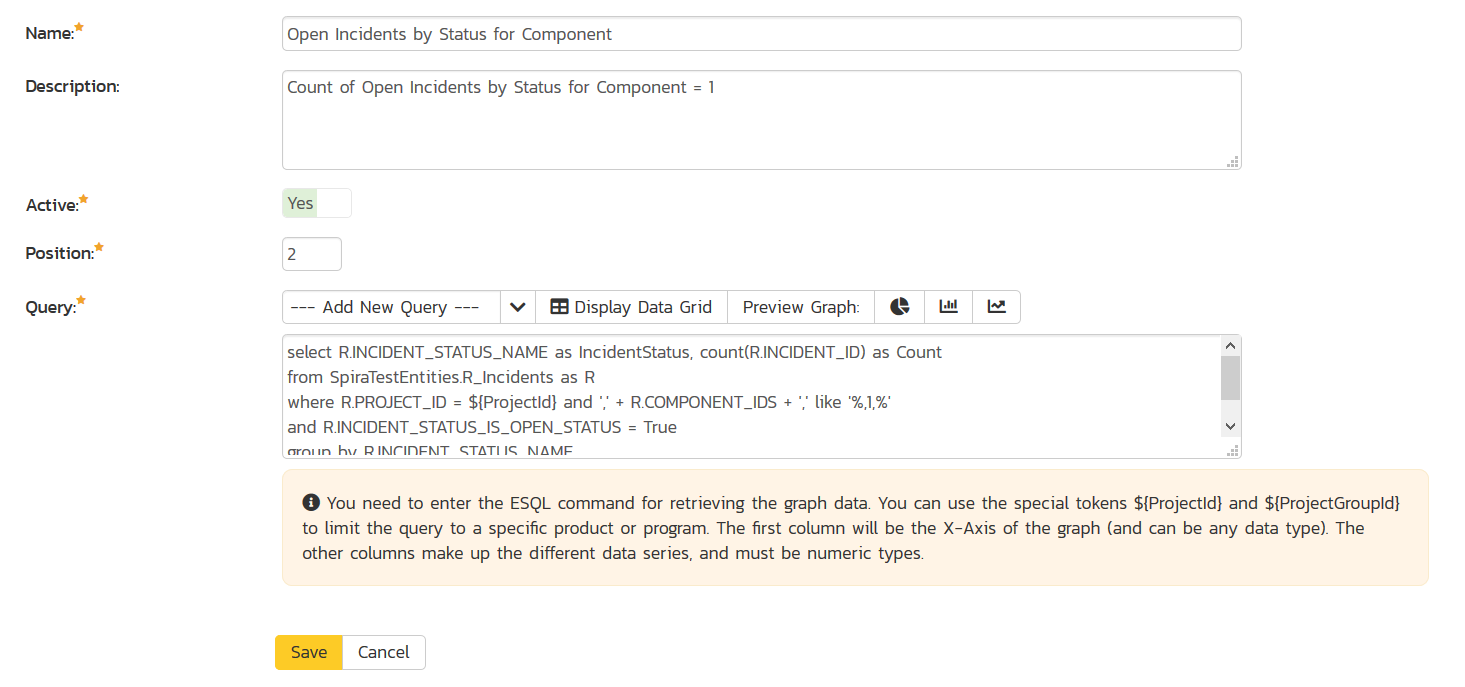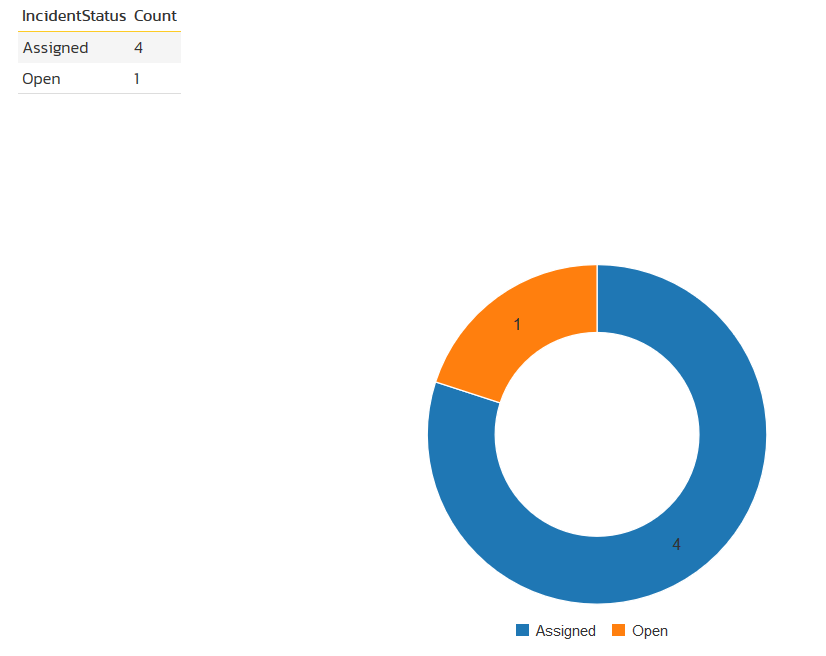## Example: Open Incidents by Status for a Component

In this example, we will show you how to create a simple donut Graph of incidents that are part of Component 1 in the current project. The graph will be a donut Graph with current incidents status as the 'axis', filtered by the specific project, component, and the IS_ACTIVE_STATUS flag set to True.

Using the option to Create a Custom Graph, we entered this information:This then gave the following data grid and donut graph:``````select R.INCIDENT_STATUS_NAME as IncidentStatus, count(R.INCIDENT_ID) as Count
from SpiraTestEntities.R_Incidents as R
where R.PROJECT_ID = \${ProjectId} and ',' + R.COMPONENT_IDS + ',' like '%,1,%'
and R.INCIDENT_STATUS_IS_OPEN_STATUS = True
group by R.INCIDENT_STATUS_NAME
``````

Which is hard-coded to filter on Component = 1

## Other Examples

To do the same thing for closed incidents, we would simply use:

``````select R.INCIDENT_STATUS_NAME as IncidentStatus, count(R.INCIDENT_ID) as Count
from SpiraTestEntities.R_Incidents as R
where R.PROJECT_ID = \${ProjectId} and ',' + R.COMPONENT_IDS + ',' like '%,1,%'
and R.INCIDENT_STATUS_IS_OPEN_STATUS = False
group by R.INCIDENT_STATUS_NAME
``````

and to get the open count by Severity (instead of status) we would use:

``````select R.SEVERITY_NAME as IncidentSeverity, count(R.INCIDENT_ID) as Count
from SpiraTestEntities.R_Incidents as R
where R.PROJECT_ID = \${ProjectId} and ',' + R.COMPONENT_IDS + ',' like '%,1,%'
and R.INCIDENT_STATUS_IS_OPEN_STATUS = True
group by R.SEVERITY_NAME
``````